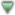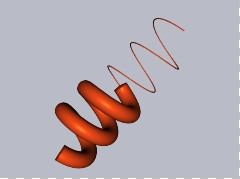###FORMULA HELP (BASICS)The shape is generated by a circle or polygon following a 3D curve.
The curve is defined with X, Y, Z as a function of time t [0:2*pi]
The width from the circle/polygon D (in mm) may be modified as a function of time.
If a polygon is used, it can be twisted by the TW function, i.e. TW=4*t
Common operators are:
+ - * / ( ) sin() cos() abs() random() round()
Allowed variables are:
t n u v w a b k r x y z d
u and v are reserved for surface generation, see tutorial
The simplest shape is a cylinder:
X = 0 Y = 0 Z = t D = 20
Actual shape dimension in mm is obtained by multiplying X, Y, Z by 12.5.
The shape is always Z translated to be above the build plane.

### ENTER FORMULA

X =
Y =
Z =
D =

S =

Show Progress

 Filename

### TEXTURE

None

Marble

Concrete

Shingle

Wood

Grass

Grid 32*32

Grid 32*4
Note: To create a 3D OBJ file with texture, first Publish and then Download the model.